Next: Conclusion Up: Random WalksMarkov Chains Previous: Differential Equations

# Markov chains

A Markov chain is a sequence of random values whose probabilities at a time interval depends upon the value of the number at the previous time. A simple example is the nonreturning random walk, where the walkers are restricted to not go back to the location just previously visited.

The controlling factor in a Markov chain is the transition probability, it is a conditional probabilty for the system to go to a particular new state, given the current state of the system. For many problems, such as simulated annealing, the Markov chain obtains the much desired importance sampling. This means that we get fairly efficient estimates if we can determine the proper transition probabilities.

Markov chains can be used to solve a very useful class of problems in a rather remarkable way. We will illustrate with the following problem: suppose we wanted to find the value of the vector x that is the solution to,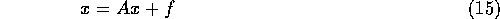where the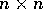matrix A, and the vector f are known. By setting up a random walk through the matrix A we can solve for any single component of x.

A little mathematics is needed to see how this would work. First lets symbolically solve (15),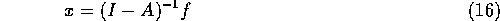This can be expanded to,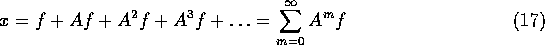Now lets suppose we have anmatrix of probabilities, P, such that,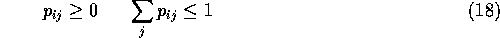and we have an array,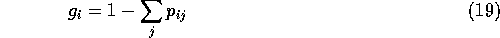further we will define,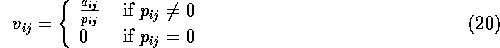P can then describe a Markov chain where the states of the chain are n integers. The element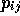gives the transition probability for the random walk to go from state i to state j. As long as g is not zero the walk will eventually terminate. The probability that the walk will terminate after state i is given by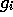.

While taking the random walk we need to accumulate the product,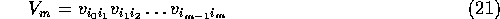and the sum,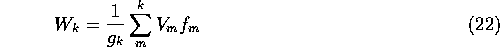The final W value is important because, its mean value (averaged over the walks that start at index i) is,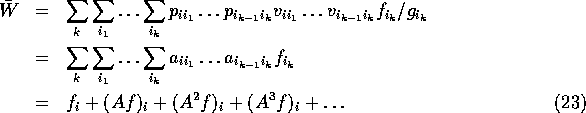Notice that the final form of (23) is exactly the ith element from equation (17).

So to solve this problem we have three major steps:

• Set up the probabilities p and g and start off the system at the index at which we want to solve for x, lets call that index i.
• Then we take a random walk until the walk terminates, accumulating the product V and the sum W.
• Then we take the average of the W values over several walks to obtain our estimate of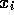.

This will work as long as equation (17) converges, this will happen if the norm of A,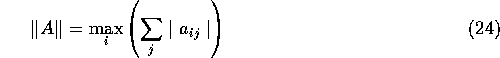is less than one (the smaller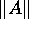is the faster the Monte Carlo estimate will converge). If the norm is larger than one, all is not lost, there is usually some manipulation that can be done to get a new matrix that has a small norm.

It turns out we can use this idea for all sorts of problems that have the same general form as (15). If we write (15) as,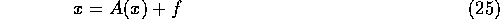and now consider A to be any linear operator that can operate on x, not just a matrix multiply. Given the appropriate operator for a given problem, we can use the above method to solve several kinds of problems. We can do a matrix inverse, i.e. solve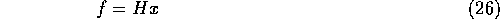if we let A = I - H. Starting out at index i, will give us row i of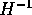.

If we restrict the chains to start at index i and end at index j, then we obtain a single element of the inverse,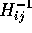. Other problems that can be solved this way include the determination of eigenvalues and eigenvectors, and integral equations of the second kind such as,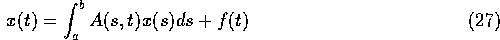Notice that equation (27) has the same kind of form as equation (25), (integration is a linear operator). If we made a discrete grid upon which we wanted to solve (27) then we could use exactly the same code that we used to solve equation (15). However in a practical application the dimension of equation (27) would be extremely large, or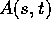would be so complicated to calculate that it is not really practical to create a giant matrix to approximate the integral. Instead we free up our random walk to apply continuously within the range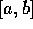. Then the system is solved with a program that otherwise looks very much like the one to solve the matrix problem.Next: Conclusion Up: Random WalksMarkov Chains Previous: Differential Equations

Skip Carter
Tue Jan 9 12:38:54 PST 1996